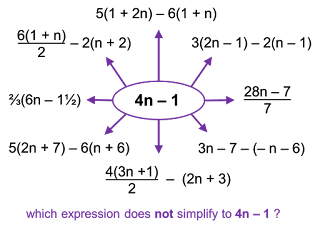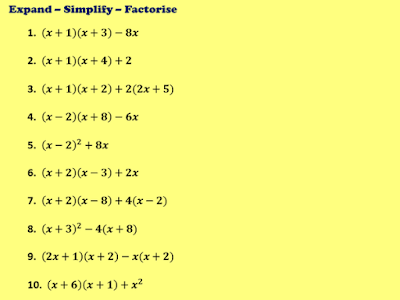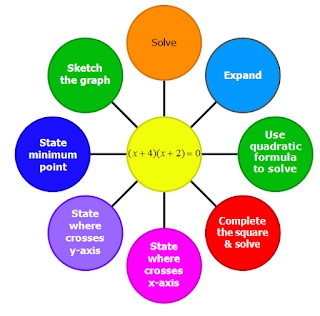## Pages

### AlgebraThis page lists recommended resources for teaching algebraic topics at Key Stage 3/4.

Huge thanks to all individuals and organisations who share teaching resources.

Quick links:  Forming Expressions  |  Function Machines  |  Simplifying Expressions  |  Expanding Single Brackets  |  Expanding Double Brackets  |  Expanding Cubics  |  Factorising (Single Brackets)  |  Rearranging Formulae  |  Substitution  |  Linear Sequences  |  Quadratic Sequences | Other Sequences  |  Linear Equations  |  Inequalities  |  Quadratic Inequalities  |  Linear Graphs  |  Quadratics  |  Trial & Improvement  |  Iteration  |  Simultaneous Equations  |  Algebraic Fractions  |  Functions  |  Graphing Functions  |  Graph Transformations  |  Algebraic Proof and Identities  |  Equation of Circle & Tangent  |  Gradient of a Curve  |  Area under a Graph |  Revision

In addition to the resources listed below, see my blog post 'Introducing Algebra' for more ideas.

Forming Expressions

Function Machines

Simplifying ExpressionsOne incorrect simplification - Median Don Steward

Expanding Single Brackets

Expanding Double Brackets
Expanding Cubics
Factorising (Single Brackets)

Rearranging Formulae

Substitution

Linear Sequences

Other SequencesSteps to Solving Equations - MAP
Linear Equations

Linear and Graphical InequalitiesIntroduction to Inequalities - projectmaths.ie

Linear Graphs
Plotting

Parallel and perpendicular

MixedFactorising Quadratics Practice from @DJUdall
Factorising

Quadratic graphs - plotting, sketching and recognising

Completing the Square

MixedQuadratic discs - Colmanweb
Trial and Improvement

Iteration
Simultaneous Equations

Algebraic Fractions

Graphing Functions
Graph Transformations

Algebraic Proof and Identities